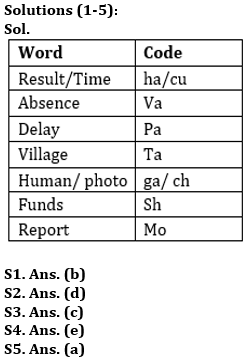Latest Banking jobs   »

# Reasoning Ability Quiz For Bank Foundation 2023 -12th April

Directions (1-5): Study the following information carefully and answer the questions given below:
In a certain code language,
‘Human photo Report Delay’ is written as ‘pa ch ga mo’,
‘Delay Village Result Time’ is written as ‘ta ha cu pa’,
‘Human photo Funds Delay’ is written as ‘ga ch sh pa’
‘Result Time Absence’ is written as ‘ha cu va’

Q1. What may be the code for ‘photo’?
(a) sh
(b) ch
(c) ha
(d) pa
(e) va

Q2. Which of the following can be the code for ‘Absence Human Report’?
(a) va mo ch
(b) ch ga mo
(c) mo ga va
(d) Either (a) or (c)
(e) None of these

Q3. ‘ha’ is the code for?
(a) Absence
(b) Village
(c) Either ‘Result’ or ‘Time’
(d) Delay
(e) None of these

Q4. Which of the following is the code for ‘Report’?
(a) ch
(b) pa
(c) cu
(d) ga
(e) None of these

Q5. ‘Time Human photo Result’ can be coded as
(a) ch ga ha cu
(b) ha cu ga sh
(c) ch ha ga mo
(d) Can’t be determined
(e) None of these

Directions (6-10): In these questions, relationship between different elements is shown in the statements. These statements are followed by two conclusions:
(a) If only conclusion I follows.
(b) If only conclusion II follows.
(c) If either conclusion I or II follows.
(d) If neither conclusion I nor II follows.
(e) If both conclusion I and II follow.

Q6. Statements: A≥Q, U>V=R, A >B≤V, U<S
Conclusion: I. B<S II. R<S

Q7. Statements: D≥Q, P>J, O>Q>J, T>D
Conclusion: I. O>T II. T>J

Q8. Statements: A≥Q, U>V=R, A >B≤V, U<S
Conclusion: I. Q≥U II. U>Q

Q9. Statements: R≥Z, C=B<Z, D≥R>O
Conclusion: I. D>B II. O<C

Q10. Statements: Q=B, K<E<B, J≥E, R≤Q
Conclusion: I. J>K II. R≤B

Direction (11-15): Study the following information carefully and answer the questions accordingly.

Q R D S T W C K G U V E J Z H I X Y A N O A M P B L G J C E D H G

Q11. How many vowels are immediately preceded and followed by the consonants?
(a) Two
(b) Four
(c) Three
(d) One
(e) Five

Q12. According to the dictionary, what will be the difference in place values of the letters which is ninth from the left end and which is sixteenth from the right end?
(a) 13
(b) 17
(c) 22
(d) 18
(e) None of these

Q13. Which of the following letter is eleventh to the right of tenth letter from the left end?
(a) A
(b) M
(c) O
(d) N
(e) None of these

Q14. How many letters are there between the letter which is fifth from the left end and tenth from the right end according to English dictionary?
(a) 4
(b) 5
(c) 2
(d) 3
(e) None

Q15. If 6th, 10th and 12th letters from the right end and from the left end are changed to their next letter according to English dictionary, then which of the following letter will be twenty fourth from the right end?
(a) K
(b) X
(c) V
(d) F
(e) None of these

SolutionsS6. Ans. (e)
Sol. I. B<S (True) II. R<S (True)

S7. Ans. (b)
Sol. I. O>T (False) II. T>J (True)

S8. Ans. (c)
Sol. I. Q≥U (False) II. U>Q (False)

S9. Ans. (a)
Sol. I. D>B (True) II. O<C (False)

S10. Ans. (e)
Sol. I. J>K (True) II. R≤B (True)

S11. Ans. (e)
Sol. GUV, VEJ, HIX, YAN, CED

S12. Ans. (d)
Sol. 9th from left end = G, 16th from right end = Y
Required difference of place values= 25 – 7 = 18

S13. Ans. (c)
Sol. Tenth from the left end – U; 11th to the right of U – O

S14. Ans. (d)
Sol. 5th from left end = T, 10th from right end = P
Thus, there are 3 letters (Q, R, S) between P and T in English alphabetic order.

S15. Ans. (c)
Sol. 24th letter from right end is U which is also 10th letter from left end, and U will become V.
Therefore, option (c) is the correct answer.## FAQs

### What is the selection process of the Bank Clerk?

The selection process of the Bank Clerk is Prelims & Mains.

#### Congratulations!Union Budget 2023-24: Free PDF